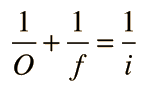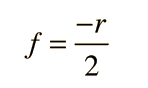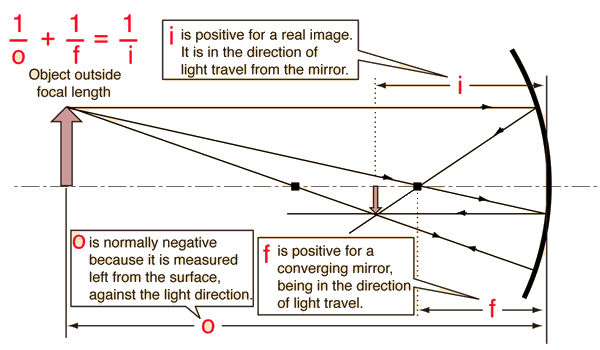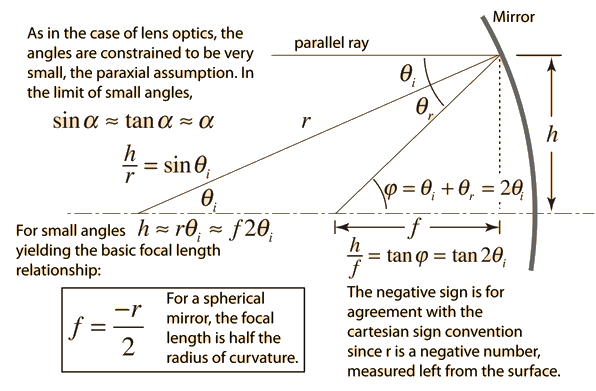# Spherical Mirror Equation

The equation for image formation by rays near the optic axis (paraxial rays) of a mirror has the same form as the thin lens equation if the cartesian sign convention is used:From the geometry of the spherical mirror, note that the focal length is half the radius of curvature:Show

As in the case of lenses, the cartesian sign convention is used here, and that is the origin of the negative sign above. The radius r for a concave mirror is a negative quantity (going left from the surface), and this gives a positive focal length, implying convergence.Index

Mirror concepts

 HyperPhysics***** Light and Vision R Nave
Go Back

# Mirror GeometryThe geometry that leads to the mirror equation is dependent upon the small angle approximation, so if the angles are large, aberrations appear from the failure of these approximations.

Index

Mirror concepts

 HyperPhysics***** Light and Vision R Nave
Go Back